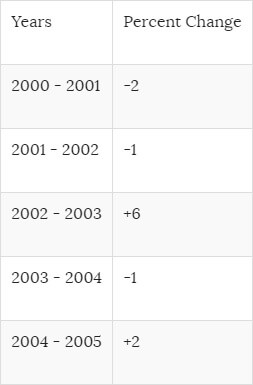# Estimation Sequence Practice Test 2

1. 18, 24
2. 20, 28
3. 21, 29
4. 21, 34
1. 68
2. 72
3. 76
4. 64
1. 64
2. 65
3. 66
4. 67
##### 4. Haley’s dad offered to pay for the team’s pizza party. The cost, including tip, would be \$4.05 per person. There were 15 team members at the party. Which of the following is the best estimate of the total bill?
1. Less than \$30
2. Between \$30 and \$40
3. Between \$40 and \$50
4. Between \$60 and \$70
5. More than \$70
1. 72
2. 120
3. 384
4. 480
1. 2
2. 1/3
3. 3
4. 1/9
1. -2
2. – 1
3. 0
4. 1
##### 8. Arrange the following numbers in order from the least to greatest: 23, 42, 60, 9, 101.
1. 23, 42, 60, 9, 101
2. 60, 9, 101, 23, 42
3. 101, 23, 60, 9, 42
4. 60, 23, 9, 101, 42
5. 9, 60, 101, 42, 23
##### 9. Put the following integers in order from least to greatest: -52, 16, -12, 14, 8, -5, 0
1. -52, 16, -12, 14, 8, -5, 0
2. 0, -5, 8, -12, 14, 16, -52
3. 0, -5, -12, -52, 8, 14, 16
4. -5, -12, -52, 0, 8, 14, 16
5. -52, -12, -5, 0, 8, 14, 16
#####10. In the year 2000, 35% of the company sales were in electronics. The table below shows how electronic sales have changed for the company over the years. Find the percent of electronics sold in 2005.
1. 2%
2. 11%
3. 39%
4. 42%
5. 47%

###### 1. D:

In this series, each number is the sum of the two preceding numbers. For example, 3 = 1 + 2, and 5 = 3 + 2. Therefore, the number following 13 must be 13 + 8 = 21, and the next number must be 21 + 13 = 34.

###### 2. A:

In this series, the number subtracted from the preceding term increases by two for each term: 108 – 4 = 104; 104 – 6 = 98; 98 – 8 = 90; 90 – 10 = 80. For the next term, subtract 12: 80 – 12 = 68. Choice A is correct.

###### 3. C:

In this series, the number added to the preceding term increases by one for each term: 41 + 3 = 44; 44 + 4 = 48; 48 + 5 = 53; 53 + 6 = 59. For the next term, add 7: 59 + 7 = 66. Choice C is correct.

###### 4. D:

To estimate, round \$4.05 to an even \$4.00, then multiply by the number of people (15). The result is \$60.

###### 5. D:

480. The series of numbers is formed by multiplying the previous number by a factor that increases by one each time:: 4*2 = 8, then 8*3 = 24, then 24*4 = 96. So the next operation is to multiply 96 by 5, resulting in 480.

###### 6. B:

1/3. Each number is found by dividing the previous one by three: 27/3 = 9, 9/3 = 3, and 3/3 = 1. The next number in the series is 1/3 = 1/3.

###### 7. A:

– 2. There are two different patterns going on here. First, we add 1, and then we subtract 2.
1 + 1 = 2, then 2 – 2 = 0. Further, 0 + 1 = 1, and 1 – 2 = – 1. Next, -1 + 1 = 0, and so the last thing to do is subtract 2, giving us 0 – 2 = -2.

###### 8. D:

When a number is raised to a power, it is multiplied by itself as many times as the power indicates. For example,23=2*2*2=8. A number raised to the power of 0 is always equal to 1, so 60 is the smallest number shown. Similarly, for the other numbers:
9=9;101=10; 42=4*4=16.

###### 9. E:

Think of the numbers as they would appear on a number line to place them in the correct order.

###### 10. C:

Let x equal the percent of electronics sold in 2005.
Then, using the series of percent changes listed in the table, we have:
x = 35 + (-2) + (-1) + (+6) + (-1) + (+2)
x = (35 + 6 + 2) + (-2 + (-1) + (-1))
x = (43) + (-4)
x = 39